SLVSCZ9A October   2015  – November 2015

PRODUCTION DATA.

1. Features
2. Applications
3. Description
4. Revision History
5. Description (continued)
6. Pin Configuration and Functions
7. Specifications
8. Parameter Measurement Information
1. 8.1 Pin Equivalent Input and Output Schematic Diagrams
2. 8.2 Timing Diagrams
9. Detailed Description
1. 9.1 Overview
2. 9.2 Functional Block Diagram
3. 9.3 Device Functional Modes
10. 10Application and Implementation
11. 11Power Supply Recommendations
12. 12Layout
13. 13Device and Documentation Support
14. 14Mechanical, Packaging, and Orderable Information

• RTQ|56
• RTQ|56

## 7 Specifications

### 7.1 Absolute Maximum Ratings

over operating free-air temperature range (unless otherwise noted)(1)
PARAMETER MIN MAX UNIT
VCC(2) Supply voltage VCC 0.3 6.0 V
IOUT Output current (dc) OUTx0 to OUTx15, x = R, G, B 30 mA
VIN(2) Input voltage SIN, SCLK, LAT, GCLK, IREF –0.3 VCC+0.3 V
VOUT(2) Output voltage SOUT –0.3 VCC+0.3 V
OUTx0 to OUTx15, x = R, G, B –0.3 11
TJ(MAX) Operating junction temperature 150 °C
TSTG Storage temperature range –55 150 °C
(1) Stresses beyond those listed under Absolute Maximum Ratings may cause permanent damage to the device. These are stress ratings only, which do not imply functional operation of the device at these or any other conditions beyond those indicated under Recommended Operating Conditions. Exposure to absolute-maximum-rated conditions for extended periods may affect device reliability.
(2) All voltage values are with respect to device ground terminal.

### 7.2 ESD Ratings

MIN MAX UNIT
V(ESD)(1) Electrostatic discharge Human body model (HBM), per ANSI/ESDA/JEDEC JS-001, all pins(2) 0 4000 V
Charged device model (CDM), per JEDEC specification JESD22-C101, all pins(3) 0 1000
(1) Electrostatic discharge (ESD) measures device sensitivity and immunity to damage caused by assembly line electrostatic discharges into the device.
(2) Level listed above is the passing level per ANSI, ESDA, and JEDEC JS-001. JEDEC document JEP155 states that 500-V HBM allows safe manufacturing with a standard ESD control process.
(3) Level listed above is the passing level per EIA-JEDEC JESD22-C101. JEDEC document JEP157 states that 250-V CDM allows safe manufacturing with a standard ESD control process.

### 7.3 Recommended Operating Conditions

At TA = –40°C to 85°C, unless otherwise noted
MIN NOM MAX UNIT
DC CHARACTERISTICS, VCC = 3 V to 5.5 V
VCC Supply voltage 3 5.5 V
VO Voltage applied to output OUTx0 to OUTx15, x = R, G, B 10 V
VIH High level input voltage SIN, SCLK, LAT, GCLK 0.7 × VCC VCC V
VIL Low level input voltage SIN, SCLK, LAT, GCLK GND 0.3 × VCC V
IOH High level output current SOUT –2 mA
IOL Low level output current SOUT 2 mA
IOLC Constant output sink current OUTx0 to OUTx15, x = R, G, B,
3 V ≤ VCC ≤ 3.6 V
20 mA
OUTx0 to OUTx15, x = R, G, B,
4 V < VCC ≤ 5.5 V
25
TA Operating free air temperature –40 85 °C
TJ Operation junction temperature –40 125 °C
AC CHARACTERISTICS, VCC = 3 V to 5.5 V(1)
FCLK(SCLK) Data shift clock frequency SCLK 25 MHz
FCLK(GCLK) Grayscale control clock frequency GCLK 33 MHz
tWH0 Pulse duration SCLK 10 ns
tWL0 SCLK 10
tWH1 GCLK 15
tWL1 GCLK 10
tSU0 Setup time SIN - SCLK↑ 2 ns
tSU1 LAT↑ - SCLK↑ 3
tSU2 LAT↓ - SCLK↑ 5
tSU3 LAT↓ (Vsync command) - GCLK↑ 2500
tSU4 The last LAT↓ for no all ‘0’ data latching to resume normal mode – GCLK↑, PSAVE_ENA bit = ‘1b’ 50 µS
tSU5 The last GCLK↑ - the 1st GCLK↑ of next line 20 ns
tH0 Hold time SCLK↑ - SIN 2 ns
tH1 SCLK↑ - LAT↑ 2
tH2 SCLK↑ - LAT↓ 13
(1) Specified by design

### 7.4 Thermal Information

THERMAL METRIC(1) TLC59581/82 UNIT
RTQ (VQFN)
56 PINS
RθJA Junction-to-ambient thermal resistance 27.4 °C/W
RθJC(top) Junction-to-case (top) thermal resistance 13.6 °C/W
RθJB Junction-to-board thermal resistance 5.5 °C/W
ψJT Junction-to-top characterization parameter 0.2 °C/W
ψJB Junction-to-board characterization parameter 5.5 °C/W
RθJC(bot) Junction-to-case (bottom) thermal resistance 0.8 °C/W

### 7.5 Electrical Characteristics

At VCC = 3.0 V to 5.5 V and TA = –40°C to 85°C, VLED = 5.0 V; Typical values are at VCC = 3.3 V, TA = 25°C (unless otherwise noted).
PARAMETER TEST CONDITIONS MIN TYP MAX UNIT
VOH Output voltage High IOH = –2 mA at SOUT VCC–0.4 VCC V
VOL Low IOL= 2 mA at SOUT 0.4 V
VLOD0 LED open detection threshold LODVTH = 00b 0.12 0.2 0.28 V
VLOD1 LODVTH = 01b 0.32 0.4 0.48
VLOD2 LODVTH = 10b 0.52 0.6 0.68
VLOD3 LODVTH = 11b 0.72 0.8 0.88
VIREF Reference voltage output RIREF = 6.2 kΩ　(1 mA target), BC = 0h, CCR/G/B = 81h 1.19 1.209 1.228 V
IIN Input current (SIN, SCLK) VIN = VCC or GND –1 1 µA
ICC0 Supply current (VCC) SIN/SCLK/LAT/GSCLK = GND, GSn = 0000h, BC = 0h,
CCR/G/B = 100h, PCHG_EN = 0, VOUTn = VCC,
RIREF = OPEN
9 11 mA
ICC1 SIN/SCLK/LAT/GSCK = GND, GSn = 0000h, BC = 4h,
CCR/G/B = 140h,VOUTn Floating, PCHG_EN = 0,
RIREF = 7.5 kΩ (Io = 10 mA target)
11 13
ICC2 SIN/SCLK/LAT = GND, GCLK = 33 MHz, TSU5 = 200 nS,
8+8 mode, GSn = FFFFh, BC = 4h, CCR/G/B = 140h,
VOUTn = 1 V when channel on, VOUTn = VCC
when channel off. PCHG_EN = 0
25 31
ICC3 SIN/SCLK/LAT = GND, GCLK = 33 MHz, TSU5 = 200 nS,
8+8 mode, GSn = FFFFh, BC = 7h, CCR/G/B = 1FFh,
VOUTn = 1 V when channel on, VOUTn = VCC
when channel off. PCHG_EN = 0
28 33
ICC4 In power save mode and PCHG_EN = 1 1 1.4
ΔIOLC0 Constant current error
(OUTx0-15, x = R/G/B)
Channel-to-channel(1) All OUTn = on, BC = 0h, CCR/G/B = 81h,
VOUTn = VOUTfix = 1 V, RIREF = 6.2 kΩ(1 mA target),
TA = 25°C, at same color grouped output of OUTR0-15,
OUTG0-15 and OUTB0-15
±1% ±3%
ΔIOLC1 Constant current error
(OUTx0-15, x = R/G/B)
Device-to-device(2) All OUTn = on, BC = 0h, CCR/G/B = 81h,
VOUTn = VOUTfix = 1 V, RIREF = 6.2 kΩ(1 mA target),
TA = 25°C, at same color grouped output of OUTR0-15,
OUTG0-15 and OUTB0-15
±1% ±2%
ΔIOLC2 Line regulation(3) VCC = 3.0 to 5.5 V, All OUTn = on, BC = 0h, CCR/G/B = 81h,
VOUTn = VOUTfix = 1 V, RIREF = 6.2 kΩ　(1 mA target)
±1 ±1.5 %/V
ΔIOLC3 Load regulation(4) All OUTn = on, BC = 0h, CCR/G/B = 81h, VOUTn = 1 to 3 V,
VOUTfix = 1 V, RIREF = 6.2 kΩ　(1 mA target)
±1 ±1.5 %/V
ΔIOLC4 Constant current error (OUTx0-15, x = R/G/B) Channel-to-channel(1) All OUTn = on, BC = 7h, CCR/G/B = 1F7h, VOUTn = VOUTfix = 1 V,
RIREF = 7.5 kΩ(25 mA target), TA = 25°C, at same color
grouped output of OUTR0-15, OUTG0-15 & OUTB0-15
±1% ±3%
ΔIOLC5 Constant current error (OUTx0-15, x = R/G/B) Device-to-device(2) All OUTn = on, BC = 7h, CCR/G/B = 1F7h, VOUTn = VOUTfix = 1 V,
RIREF = 7.5 kΩ(25 mA target), TA = 25°C, at same color
grouped output of OUTR0-15, OUTG0-15 and OUTB0-15
±1% ±2%
ΔIOLC6 Line regulation(3) VCC = 3.0 to 5.5 V, All OUTn = on, BC = 7h, CCR/G/B = 1F7h,
VOUTn = VOUTfix = 1 V, RIREF = 7.5 kΩ　(25 mA target)
±1 ±1.5 %/V
ΔIOLC7 Load regulation(4) All OUTn = on, BC = 7h, CCR/G/B = 1F7h, VOUTn = 1 to 3 V,
VOUTfix = 1 V, RIREF = 7.5 kΩ　(25 mA target)
±1 ±1.5 %/V
TTSD Thermal shutdown threshold(5) 160 170 180 °C
THYS Thermal shutdown hysterisis 10 °C
VISP(in) IREF resistor short protection threshold 0.15 0.195 V
VISP(out) IREF resistor short-protection release threshold 0.325 0.4 V
RPDWN Pull-down resistor LAT 250 500 750
RPUP Pull-up resistor GCLK 250 500 750
Vknee (5) Knee voltage (OUTX 0~15), X = R/G/B All OUTn = on, BC = 4h, CCR/G/B = 137h, RIREF = 7.5 kΩ. (Io = 10 mA target) 0.32 0.35 V
(1) The deviation of each output in same color group (OUTR0~15 or OUTG0~15 or OUTB0~15) from the average of same color group constant current. The deviation is calculated by the formula. (X = R or G or B, n = 0~15)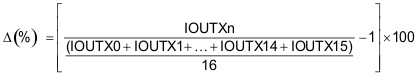spacer
(2) The deviation of the average of constant-current in each color group from the ideal constant-current value. (X = R or G or B) :Ideal current is calculated by the following equation:spacer
(3) Line regulation is calculated by the following equation. (X = R or G or B, n = 0~15):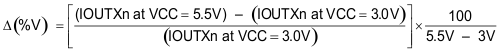spacer
(4) Load regulation is calculated by the following equation. (X = R or G or B, n = 0~15):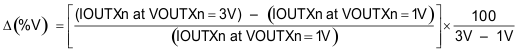spacer
(5) Specified by design.

### 7.6 Typical Characteristics

VCC = 3.3 V and TA = 25°C, unless otherwise noted.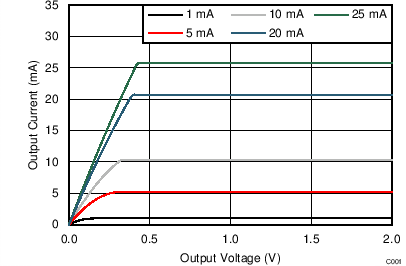VCC = 5 V CCR/G/B = 1FFh, BC = 0
Figure 1. Output Current vs Output Voltage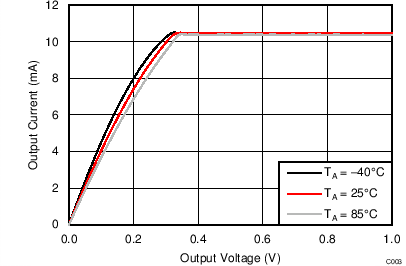VCC = 5 V Temperature Changing CCR/G/B = 1FFh, BC = 0
Figure 3. Output Current vs Output Voltage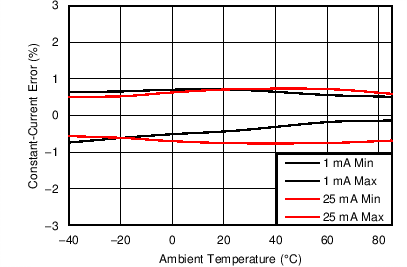VCC = 5 V VOUTXn = 0.8 V CCR/G/B = 1FFh, BC = 0
Figure 5. Constant-Current Error (CH-to-CH) vs Temperature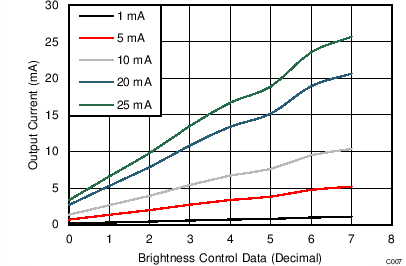VCC = 5 V VOUTXn = 0.8 V CCR/G/B = 1FFh
Figure 7. Brightness Control (BC) vs Output Current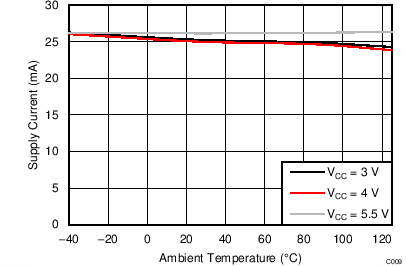VOUTXn = 0.8 V CCR/G/B = 137h, BC = 4, GCLK = 33 MHz GSXn = FFFFh, RIREF = 7.5 kΩ (10-mA target)
Figure 9. Supply Current (Icc) vs Temperature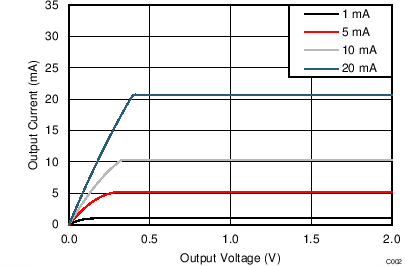VCC = 3.3 V CCR/G/B = 1FFh, BC = 0
Figure 2. Output Current vs Output Voltage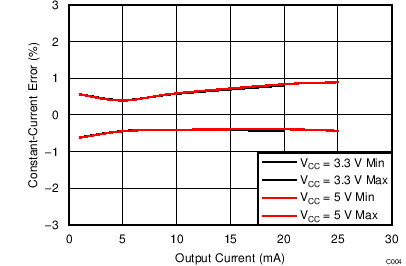VOUTXn = 0.8 V CCR/G/B = 1FFh, BC = 0
Figure 4. Constant Current Error (CH-to-CH) vs Output Current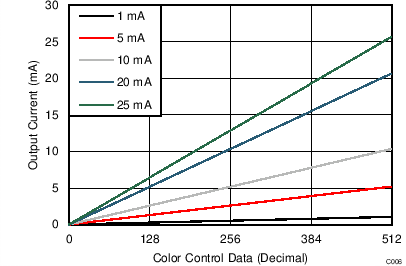VCC = 5 V VOUTXn = 0.8 V BC = 7
Figure 6. Color Control (CC) vs Output Current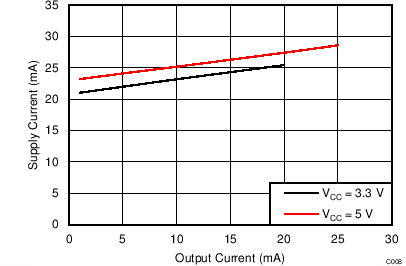VOUTXn = 0.8 V GCLK = 33 MHz, GSXn = FFFFh CCR/G/B = 1FFh, BC = 0
Figure 8. Supply Current (Icc) vs Output Current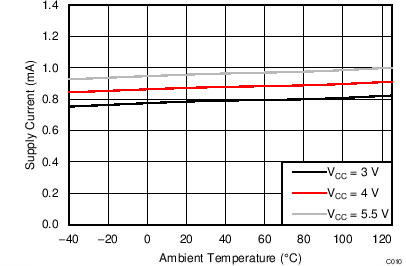VOUTXn = 0.8 V CCR/G/B = 137h, BC = 4 GCLK = GND, GSXn = 0h
Figure 10. Supply Current in Power Save Mode (Icc)
vs Temperature